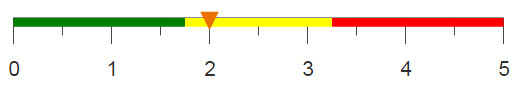# Transforming dates from text to integers

Sometimes dates are stored in a variety of text formats for display purposes, but in order to operate on these dates using 1010data functions, they need to be transformed to 1010data's standard YYYYMMDD integer format.

## Difficulty## Objective

You have a column containing the date for every row in your table, but the dates are stored as text instead of integers. You want to transform each date into 1010data's standard integer format (YYYYMMDD).

## Solution

Transform a date stored as a string in the form MMDDYYYY (e.g., 01042015)

```<willbe name="new_date"
value="int(splice(strextract(date_column;5;4)
strextract(date_column;1;2) strextract(date_column;3;2);''))"
format="type:date"/>```

Transform a date stored as a string in the form MM-DD-YYYY (e.g., 01-04-2015)

```<willbe name="new_date"
value="int(splice(strpick(date_column;'-';3)
strpick(date_column;'-';1) strpick(date_column;'-';2);''))"
format="type:date"/>```

Transform a date stored as a string in the form MM/DD/YYYY (e.g., 01/04/2015)

```<willbe name="new_date"
value="int(splice(strpick(date_column;'/';3)
strpick(date_column;'/';1) strpick(date_column;'/';2);''))"
format="type:date"/>```

Transform a date stored as a string in the form MM/DD/YY (e.g., 01/04/15)

```<willbe name="new_date"
value="int(splice(splice('20' strpick(date_column;'/';3);'')
strpick(date_column;'/';1) strpick(date_column;'/';2);''))"
format="type:date"/>```

Transform a date stored as a string in the form M/D/YYYY (e.g., 1/4/2015)

```<willbe name="new_date"
value="int(splice(strpick(date_column;'/';3)
if(width(strpick(date_column;'/';1))=1;
splice('0' strpick(date_column;'/';1);'');
strpick(date_column;'/';1))
if(width(strpick(date_column;'/';2))=1;
splice('0' strpick(date_column;'/';2);'');
strpick(date_column;'/';2));''))"
format="type:date"/>```

Transform a date stored as a string in the form M/D/YY (e.g., 1/4/15)

```<willbe name="new_date"
value="int(splice(splice('20' strpick(date_column;'/';3);'')
if(width(strpick(date_column;'/';1))=1;
splice('0' strpick(date_column;'/';1);'');
strpick(date_column;'/';1))
if(width(strpick(date_column;'/';2))=1;
splice('0' strpick(date_column;'/';2);'');
strpick(date_column;'/';2));''))"
format="type:date"/>```

Transform a date stored as a string in the form DD-Mon-YY (e.g., 04-Jan-14)

```<willbe name="date_reorder"
value="splice(strdrop(strtake(date_column;'-';2);'-';1)
strtake(date_column;'-';1) strdrop(date_column;'-';2);'-')"/>
<willbe name="date_replace" value="repstr(date_reorder;
'Jan' '01' 'Feb' '02' 'Mar' '03' 'Apr' '04' 'May' '05' 'Jun' '06'
'Jul' '07' 'Aug' '08' 'Sept' '09' 'Oct' '10' 'Nov' '11' 'Dec' '12')"/>
<willbe name="new_date"
value="int(splice(splice('20' strpick(date_replace;'-';3);'')
strpick(date_replace;'-';1) strpick(date_replace;'-';2);''))"
format="type:date"/>
<colord hide="date_reorder,date_replace"/>```

## Discussion

In 1010data, dates are stored internally as 8-digit integers with the form YYYYMMDD. For instance, January 4, 2015, would be stored in 1010data as 20150104. Though storing dates this way is more efficient in terms of processing speeds, dates are not commonly presented in this way. 1010data uses display formats to show dates in more familiar forms. For example, 20150104 could be displayed as 01/04/15 using the date format, or as 01/04/2015 using the date4y format. Regardless of how this date is displayed, it is still stored as the integer 20150104.

However, sometimes dates are not loaded into 1010data as integers. Sometimes, dates are loaded as text values, and the forms that these strings can take may vary. Some examples are:
• 1/4/15
• 01042015
• 01-04-2015
• 1/4/2015
Dates stored as text are not recognized by 1010data as dates and can’t be used in any of the date-related functions. For instance, the function year(X) returns the year portion of a date value. However, if you try to provide this function with a date stored as text, you’ll get an error.
Note: You can find out the data type of the column containing your date values by clicking the Show Information icon () at the top of the column in the 1010data Trillion-Row Spreadsheet®.

This solution provides some simple manipulations to transform various text formats into the proper integer type so that they can be recognized and processed correctly by 1010data. All of the transformations assume that the text column in which the dates are stored is named date_column, although that name is arbitrary and purely for example.

## Common errors

eslc{} An error was trapped at subprocess (nj3023/13015). The error message was: While evaluating computed column new_date the following error occurred: Internal (type) error
Remember that all of these date formats are stored as text values. If you try to change dates saved as integers, you will get this error. To avoid this error, double check the data type of the values in the column by clicking the Show Information icon () at the top of the column.

## Further reading

If you would like to learn more about the functions and operations discussed in this recipe, click on the links below:

splice(X;Y)

strextract(X;P;N)

strpick(X;Y;I)

strtake(X;Y;I)

if(C1;R1;C2;R2;...;D)